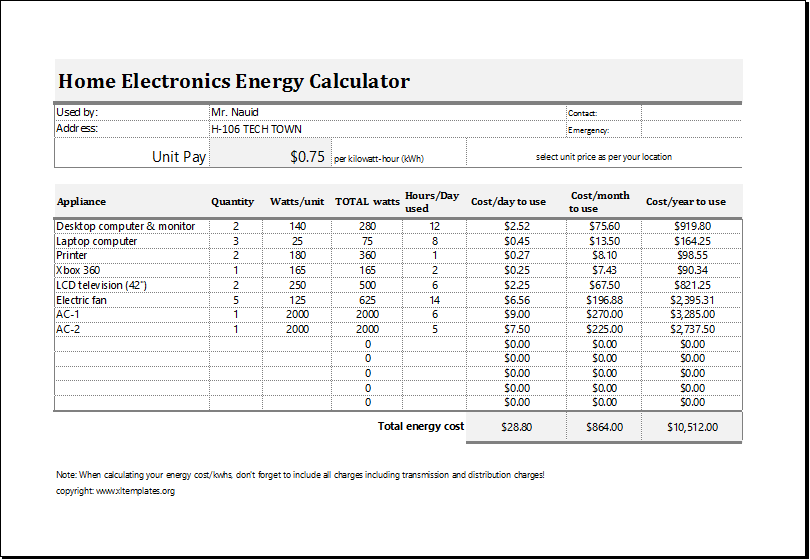# Electric Energy Cost Calculator

We live in a world that runs on energy, and not the energy that we humans live on but the energy which is generated by various powerhouses and various dams and mills. This power is given to various sectors (residential and commercial) and then the power is used to complete various tasks and various everyday things.

Because the energy that human beings use isn’t free and there is a cost that is to be paid by the people to the power companies, one must make use of an energy cost calculator which helps in knowing how much energy are we consuming and what is the worth of that energy and how much money is to be paid by the people.

Many people want to know how much the house appliances consume energy. The electricity cost calculator bill helps them get the necessary information regarding the bill. The total energy usage at home or at the office can be very helpful.

The calculator helps the person calculate total energy consumed in kilowatt per hour and saving in energy by substituting the high energy-consuming devices by energy-efficient devices. The purpose of using the calculator is to get a precise reading.

## The Calculator

This energy cost calculator is one of the easiest ways of calculating the cost of the energy that is being consumed by the various houses, businesses and according to that calculation, one can always set a budget.

This energy cost calculator also helps in maintaining a budget for the household or work and helps in saving money and etc. this energy expense calculator works like this, one chooses the number of appliances that are running the household and then type in the power in watts that they consume, then one enters the number of hours that they run on an average on a daily basis and then hits enter. VOILA, one knows how much energy is being used and what the cost that occurs because of these appliances is.

## Benefits of energy cost calculator:

There are plenty of benefits the cost calculator comes with. Some of the key benefits are:

1. The cost calculator enables the person to know which appliance consumes more electricity. He can then opt for a better substitute in order to save energy.
2. In order to control the electricity bill, a person should be able to perform the cost calculator. This enables him to make the budget of the house according to it.
3. The cost calculator gives a clear picture of the cost the user will have to incur. This becomes a motivation for a user to save energy.
4. The user can also see the consumption of energy per day, month, and per year.

The electricity cost calculator has pre-defined formulas in it. There are different details that the calculator requires the user to provide. Once the user provides all these details, the calculations are performed by the calculator itself.

The calculator obtains the information such as the name of appliances being used, the total power consumed by those appliances, the number of hours the appliances are used, the cost of the electricity per unit, and a lot more. On the basis of information collected by the calculator, the necessary results are provided by the calculator.

## How to Use?

To use this sheet you need to know the power used by each appliance. It is not a difficult task as you can use the appliance handbook or just look at someplace around it. Enter the number given in units WATTS. Count the number of appliances being used to enter the next column. Set unit cost at your place of living and it is all set to calculate the cost for you. The cost is calculated per day, per month, and on a yearly basis.

## The Template

The electricity energy cost calculator is usually prepared in MS Excel. It is easier to make the calculator in Excel since the use of formulas in Excel is easy. Excel has many default formulas due to which the user finds it easy to use this software for calculation. The calculator template can also be used in order to save time and money.

Preview